ICSE Class 10 Compound Interest Solution New Pattern

## ICSE Class 10 Compound Interest Solution New Pattern By Clarify Knowledge

ICSE Class 10 Compound Interest Solution New Pattern 2022

Question 1

The difference between simple interest and compound interest on a certain sum is54.40 for 2 years at 8 per cent per annum. Find the sum.Solution 1

Let principal (P) = x

R = 8%

T = 2 years

Given, CI = SI = 54.40

Thus, principal sum = Rs. 8500Question 2

A sum of money, invested at compound interest, amounts to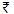19,360 in 2 years and to23,425.60 in 4 years. Find the rate per cent and the original sum of money.Solution 2

(for 2 years) A = Rs. 19360

T = 2 years

Let P = X

...(1)

A (for 4 years) = Rs. 23425.60

...(2)

(2) (1)

Form (1), we have

Thus, sum = Rs. 16000Question 3

A sum of money lent out at C.I. at a certain rate per annum becomes three times of itself in 8 years. Find in how many years will the money becomes twenty-seven times of itself at the same rate of interest p.a.Solution 3

Let principal = x, A = 3x, T = 8 years, R = ?

Case I,

Case II,

P = x, A = 27x, T = ?

From (1) and (2)

Hence, time = 24 years.Question 4

On what sum of money will compound interest (payable annually) for 2 years be the same as simple interest on9,430 for 10 years, both at the rate of 5 per cent per annum?Solution 4

P = Rs. 9430

R = 5%

R = 10 years

SI =

Let sum = x

CI = 4715, T = 2 years, Rs= 5%

CI = A - P

Thus principal from = Rs. 46,000Question 5

Kamal and Anand each lent the same sum of money for 2 years at 5% at simple interest and compound interst respectively. Anand recived15 more than Kamal. Find the amount of money lent by each and the interest received.Solution 5

Let principal = Rs. 100, R = 5% T = 2 years

For Kamal, SI =

For Anand,CI =Difference of CI and SI =

When difference is Rs. , then principal = Rs. 100

If difference is 1, principal = 100 4

If difference is Rs, 15, principal = 100 15 = Rs. 6000

For kamal, interest =

For Anand, interest =

Question 6

Simple interest on a sum of money for 2 years at 4% is450. Find compound interest of the same sum and at the same rate for 2 years.Solution 6

SI = Rs. 450

R = 4%

T = 2 years

P = ?

Now, P = 5625, R = 4%, T = 2 years

A =

CI = A - P = 6084 - 5625

= Rs. 459Question 7

Simple interest on a certain sum of money for 4 years at 4% per annum exceeds the compound interest on the same sum for 3 years at 5 per cent per annum by228. Find the sum.Solution 7

Let principal (P), R = 4%, T = 4 years

Given: SI - CI = Rs. 228

Thus, Principal = Rs. 96000Question 8

Compound interest on a certain sum of money at 5% per annum for two years is246. Calculate simple interest on the same sum for 3 years at 6% per annum.Solution 8

CI = Rs. 246, R = 5%, T = 2 years

CI = A - P

Now, P = Rs. 2400, R = 6%, T = 3 years

Question 9

A certain sum of money amounts to Rs. 23,400 in 3 years at 10% per annum simple interest. Find the amount of the same sum in 2 years and at 10% p.a. compound interest.Solution 9

Let the sum (principle) = x

Given Amount = 23400, R = 10% and T = 3 years

Amount = Principle + Interest

23400 == 18000

Principle = 18000

Now,

Principle = `18000, r = 10% and n = 2 years

The amount of the same sum in 2 years and at 10% p.a. compound interest is 21780.Question 10

Mohit borrowed a certain sum at 5% per annum compound interest and cleared this loan by paying Rs. 12,600 at the end of the first year and Rs. 17,640 at the end of the second year. Find the sum borrowed.Solution 10

## Chapter 3 - Compound Interest (Using Formula) Exercise Ex. 3(C)

Question 1

If the interest is compounded half-yearly, calculate the amount when principal is Rs7,400; the rate of interest is 5% per annum and the duration is one year.Solution 1

Given: P=Rs7,400; r=5% p.a. and n= 1year

Since the interest is compounded half-yearly,

Then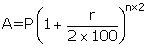Question 2

Find the difference between the compound interest compounded yearly and half-yearly on Rs10,000 for 18 months at 10% per annum.Solution 2

(i)When interest is compounded yearly

Given: P=Rs10,000; n=18months=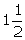year and r=10%p.a.

For 1year

For1/2 year

P=Rs11,000;n= 1/2 year and r=10%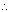C.I.= Rs11,550 - Rs10,000= Rs1,550

(ii)When interest is compounded half-yearly

P=Rs10,000; n=year and r=10%p.a.C.I.= Rs11,576.25 - Rs10,000=Rs1,576.25Difference between both C.I.= Rs1,576.25 - Rs1,550

= Rs26.25 Ans.Question 3

A man borrowed Rs.16,000 for 3 years under the following terms:

20% simple interest for the first 2 years.

20% C.I. for the remaining one year on the amount due after 2 years, the interest being compounded half-yearly.

Find the total amount to be paid at the end of the three years.Solution 3

For the first 2 years

Amount in the account at the end of the two years is Rs.22,400.

For the remaining one year

The total amount to be paid at the end of the three years is Rs.27,104.Question 4

What sum of money will amount to Rs.27,783 in one and a half years at 10% per annum compounded half yearly?Solution 4

The sum of Rs.24,000 amount Rs.27,783 in one and a half years at 10% per annum compounded half yearly.Question 5

Ashok invests a certain sum of money at 20% per annum, compounded yearly. Geeta invests an equal amount of money at the same rate of interest per annum compounded half-yearly. If Geeta gets Rs33 more than Ashok in 18 months, calculate the money invested.Solution 5

(i)For Ashok(interest is compounded yearly)

Let P=Rs y; n=18months=year and r=20%p.a.

For 1year

For1/2 year

P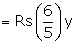;n= ½ year and r=20%

(ii)For Geeta(interest is compounded half-yearly)

P=Rs y; n=year and r=20%p.a.

According to questionMoney invested by each person=Rs3,000 Ans.Question 6

At what rate of interest per annum will a sum of Rs.62,500 earn a compound interest of Rs.5,100 in one year? The interest is to be compounded half yearly.Solution 6

The rate of interest is 8%.Question 7

In what time will Rs1,500 yield Rs496.50 as compound interest at 20% per year compounded half-yearly?Solution 7

Given: P=Rs1,500; C.I.=Rs496.50 and r=20%

Since interest is compounded semi-annually

Then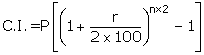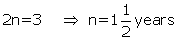Ans.Question 8

Calculate the C.I. on Rs3,500 at 6% per annum for 3years, the interest being compounded half-yearly.

Do not use mathematical tables. Use the necessary information from the following:

(1.06)3 =1.191016; (1.03)3 = 1.092727

(1.06)6 =1.418519; (1.03)6 = 1.194052Solution 8

Given: P=Rs 3,500; r=6% and n= 3years

Since interest is being compounded half-yearly

ThenAns.Question 9

Find the difference between compound interest and simple interest on Rs12,000 and inyears at 10% compounded yearly.Solution 9

Given: P=Rs12,000; n=years and r= 10%

To calculate C.I.

For 1 year

P=Rs 12,000; n=1 year and r=10%

For next 1/2 year

P=Rs 13,200; n= 1/2 year and r=10%C.I. = Rs 13,860 - Rs 12,000= Rs 1,860Difference between C.I. and S.I

= Rs 1,860 - Rs 1,800=Rs 60 Ans.Question 10

Find the difference between compound interest and simple interest on Rs12,000 and inyears at 10% compounded half-yearly.Solution 10

Given: P=Rs12,000; n=years and r= 10%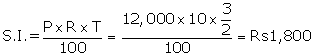To calculate C.I.(compounded half-yearly)

P=Rs12,000;n=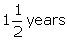and r=10%C.I.- Rs12,000= Rs1,891.50Difference between C.I. and S.I

=Rs1,891.50 - Rs1,800=Rs91.50 Ans.

## Chapter 3 - Compound Interest (Using Formula) Exercise Ex. 3(D)

Question 1

The cost of a machine is supposed to depreciate each year at 12% of its value at the beginning of the year. If the machine is valued at Rs44,000 at the beginning of 2008, find its value :

(i)at the end of 2009.

(ii)at the beginning of 2007.Solution 1

Cost of machine in 2008 = Rs44,000

Depreciation rate=12%

(i)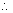Cost of machine at the end of 2009

(ii) Cost of machine at the beginning of 2007(P)

Question 2

The value of an article decreases for two years at the rate of 10% per year and then in the third year it increases by 10%. Find the original value of the article, if its value at the end of 3 years is Rs.40,095.Solution 2

Let x be the value of the article.

The value of an article decreases for two years at the rate of 10% per year.

The value of the article at the end of the 1st year is

X - 10% of x = 0.90x

The value of the article at the end of the 2nd year is

0.90x - 10% of (0.90x) = 0.81x

The value of the article increases in the 3rd year by 10%.

The value of the article at the end of 3rd  year is

0.81x + 10% of (0.81x) = 0.891x

The value of the article at the end of 3 years is Rs.40,095.

The original value of the article is Rs.45,000.Question 3

According to a census taken towards the end of the year 2009, the population of a rural town was found to be 64,000. The census authority also found that the population of this particular town had a growth of 5% per annum. In how many years after 2009 did the population of this town reach 74,088 ?Solution 3

Population in 2009 (P) = 64,000

Let after n years its population be 74,088(A)

Growth rate= 5% per annum

Ans.Question 4

The population of a town decreased by 12% during 1998 and then increased by 8% during 1999. Find the population of the town, at the beginning of 1998, if at the end of 1999 its population was 2,85,120.Solution 4

Let the population in the beginning of 1998 = P

The population at the end of 1999 = 2,85,120(A)

r1 = - 12% and r2 = +8%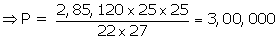Ans.Question 5

A sum of money, invested at compound interest, amounts to Rs 16,500 in 1 year and to Rs19,965 in 3 years. Find the rate per cent and the original sum of money invested.Solution 5

Let sum of money be Rs P and rate of interest= r%

Money after 1year= Rs16,500

Money after 3years=Rs19,965

For 1year

For 3years

Divide eqn (2) by eqn (1)

On comparing, we get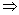r= 10% Ans.

Put value of r in eqn (1)AnsQuestion 6

The difference between C.I. and S.I. on Rs7,500 for two years is Rs12 at the same rate of interest per annum. Find the rate of interest.Solution 6

Given: P = Rs 7,500 and Time(n)= 2 years

Let rate of interest = y%

Given: C.I. - S.I. = Rs 12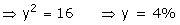Ans.Question 7

A sum of money lent out at C.I. at a certain rate per annum becomes three times of itself in 10years. Find in how many years will the money become twenty-seven times of itself at the same rate of interest p.a.Solution 7

Let Principal be Rs y and rate= r%

According to 1st condition

Amount in 10 years = Rs 3y

According to 2nd condition

Let after n years amount will be Rs 27y

Question 8

Mr. Sharma borrowed a certain sum of money at 10% per annum compounded annually. If by paying Rs.19,360 at the end of the second year and Rs.31,944 at the end of the third year he clears the debt; find the sum borrowed by him.Solution 8

At the end of the two years the amount is

Mr. Sharma paid Rs.19,360 at the end of the second year.

So for the third year the principal is A1 - 19,360.

Also he cleared the debt by paying Rs.31,944 at the end of the third year.

Mr. Sharma borrowed Rs.40,000.Question 9

The difference between compound interest for a year payable half-yearly and simple interest on a certain sum of money lent out at 10% for a year is Rs15. Find the sum of money lent out.Solution 9

Let sum of money be RS y

To calculate S.I.

To calculate C.I.(compounded half-yearly)

Question 10

The ages of Pramod and Rohit are 16 years and 18 years respectively. In what ratio must they invest money at 5% p.a. compounded yearly so that both get the same sum on attaining the age of 25 years?Solution 10

Let Rs.x and Rs.y be the money invested by Pramod and Rohit respectively such that they will get the same sum on attaining the age of 25 years.

Pramod will attain the age of 25 years  after 25 - 16 = 9 years

Rohit will attain the age of 25 years  after 25 -18 = 7 years

Pramod and Rohit should invest in 400:441 ratio respectively such that they will get the same sum on attaining the age of 25 years.

## Chapter 3 - Compound Interest (Using Formula) Exercise Ex. 3(E)

Question 1

Simple interest on a sum of money for 2 years at 4% is Rs.450. Find compound interest on the same sum and at the same rate for 1 year, if the interest is reckoned half yearly.Solution 1

1st case

Given: S.I. = Rs 450;Time= 2 years and Rate = 4%

2nd case(compounded half-yearly)

P = Rs.5,625;n= 1 year and r = 4%

Question 2

Solution 2

For 2years

For ½ year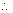C.I. = A - P = Rs.13,721 - Rs.10,800 = Rs.2,921 Question 3

The value of a machine, purchased two years ago, depreciates at the annual rate of 10%. If its present value is Rs.97,200, find:

i.  Its value after 2 years.

ii.  Its value when it was purchased.Solution 3

(i) Present value of machine(P) =  Rs.97,200

Depreciation rate = 10%

=Rs.78732

(ii) Present value of machine(A) = Rs.97,200

Depreciation rate = 10% and time = 2 years

To calculate the cost 2 years ago

Question 4

Anuj and Rajesh each lent the same sum of money for 2 years at 8% simple interest and compound interest respectively. Rajesh received Rs.64 more than Anuj. Find the money lent by each and interest received.Solution 4

Let the sum of money lent by both Rs.y

For Anuj

P = Rs.y ;rate = 8% and time = 2 years

For Rajesh

P = Rs.y ;rate = 8% and time = 2 years

Given :  C.I. - S.I. = Rs.64

Question 5

Calculate the sum of money on which the compound interest (payable annually) for 2 years be four times the simple interest on Rs.4,715 for 5 years, both at the rate of 5% per annum.Solution 5

Given: Principal = Rs.4,715;time = 5 years and rate= 5% p.a.

Then C.I. = Rs.1,178.75 x 4 = Rs.4,715

Time = 2 years and rate = 5%

Question 6

A sum of money was invested for 3 years, interest being compounded annually. The rates for successive years were 10%, 15% and 18% respectively. If the compound interest for the second year amounted to Rs.4,950, find the sum invested.Solution 6

Given: C.I. for the 2nd year = Rs.4,950 and rate = 15%

Then amount at the end of 2nd year= Rs.33,000

For first 2years

A = Rs.33,000; r1 =10%

The sum invested is Rs.30,000.Question 7

A sum of money is invested at 10% per annum compounded half yearly. If the difference of amounts at the end of 6 months and 12 months is Rs.189, find the sum of money invested.Solution 7

Let the sum of money be Rs.y

and rate = 10% p.a. compounded half yearly

For first 6months

For first 12 months

Given: The difference between the above amounts = Rs.189

y = 3600Question 8

Rohit borrows Rs.86,000 from Arun for two years at 5% per annum simple interest. He immediately lends out this money to Akshay at 5% compound interest compounded annually for the same period. Calculate Rohit's profit in the transaction at the end of two years.Solution 8

P = Rs.86,000;time = 2 years and rate = 5% p.a.

To calculate S.I.

To calculate C.I.

Profit = C.I. - S.I. = Rs.8,815 - Rs.8,600 = Rs.215Question 9

The simple interest on a certain sum of money for 3 years at 5% per annum is Rs.1,200. Find the amount and the compound interest due on this sum of money at the same rate and after 2 years. Interest is reckoned annually.Solution 9

Let Rs.x be the sum of money.

Rate = 5 % p.a. Simple interest = Rs.1,200, n = 3years.

The amount due and the compound interest on this sum of money at the same rate and after 2 years.

P = Rs.8,000;rate = 5% p.a., n = 3 years

The amount due after 2 years is Rs.8,820 and the compound interest is Rs.820.Question 10

Nikita invests Rs.6,000 for two years at a certain rate of interest compounded annually. At the end of first year it amounts to Rs.6,720. Calculate:

(a) The rate of interest.

(b) The amount at the end of the second year.Solution 10

Let x% be the rate of interest.

P = Rs.6,000, n = 2 years, A = Rs.6,720

1. For the first year

The rate of interest is x% = 12%.

1. The amount at the end of the second year.

The amount at the end of the second year = Rs.7,526.40

## Chapter 3 - Compound Interest (Using Formula) Exercise Ex. 3(A)

Question 1

Find the amount and the compound interest on Rs12,000 in 3years at 5% compounded annually.Solution 1

Given : P= Rs12,000; n=3years and r=5%

Amount=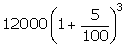==Rs13,891.50 Ans.C.I. =RS13,891.50 - Rs12,000

= Rs1,891.50 Ans.Question 2

Calculate the amount of Rs15,000 is lent at compound interest for 2years and the rates for the successive years are 8% and 10% respectively.Solution 2

Given : P= Rs15,000; n=2years; r1 =8% and r2 =10%

Amount==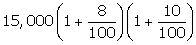=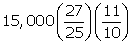=Rs17,820 Ans.Question 3

Calculate the compound interest accrued on Rs6,000 in 3years, compounded yearly, if the rates for the successive years are 5%, 8% and 10% respectively.Solution 3

Given : P=Rs6,000; n= 3years; r1= 5%; r2= 8% and r3 =10%

Amount=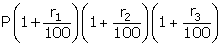==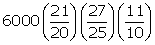=Rs7,484.40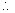C.I. = Rs7,484.40 - Rs6,000 = Rs1,484.40 Ans.Question 4

What sum of money will amount to Rs5,445 in 2years at 10% per annum compound interest?Solution 4

Given : Amount= Rs5,445; n= 2years and r = 10%A=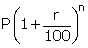5,445=5,445=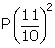P=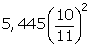=Rs4,500 Ans.Question 5

On what sum of money will the compound interest for 2years at 5% per annum amount to Rs768.75?Solution 5

Given : C.I.= Rs768.75; n= 2years and r = 5%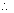A=A=A==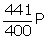A - P =C.I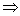- P=Rs768.75=Rs768.75P=Rs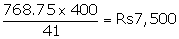Ans.Question 6

Find the sum on which the compound interest for 3years at 10% per annum amounts to Rs1,655.Solution 6

Given : C.I.= Rs1,655; n= 3years and r = 10%A=A=A=A - P =C.I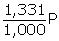- P=Rs1,655=Rs1,655P=RsAns.Question 7

What principal will amount to Rs9,856 in two years, if the rates of interest for successive years are 10% and 12% respectively?Solution 7

Given : Amount =Rs9,856; n=2years; r1 =10% and r2 =12%

Ans.Question 8

On a certain sum, the compound interest in 2 years amounts to Rs.4,240. If the rate of interest for the successive years is 10% and 15% respectively, find the sum.Solution 8

The sum is Rs.16,000Question 9

At what per cent per annum will Rs.6,000 amount to Rs.6,615 in 2 years when interest is compounded annually?Solution 9

At 5% per annum the sum of Rs.6,000 amounts to Rs.6,615 in 2 years when the interest is compounded annually.Question 10

At what rate per cent compound interest, does a sum of money become 1.44 times of itself in 2years?Solution 10

Let Principal = Rs y

Then Amount= Rs 1.44y

n= 2years

Question 11

At what rate per cent will a sum of Rs. 4,000 yield Rs. 1,324 as compound interest in 3 years?Solution 11

Question 12

A person invests Rs5,000 for three years at a certain rate of interest compounded annually. At the end of two years this sum amounts to Rs6,272. Calculate :

(i)the rate of interest per annum.

(ii)the amount at the end of the third year.Solution 12

Given: P=Rs5,000; A=Rs6,272 and n= 2years

(i)

(ii) Amount at the third year

Question 13

In how many years will Rs7,000 amount to Rs9,317 at 10% per annum compound interest?Solution 13

Given : P=Rs7,000; A=Rs9,317 and r= 10%

Question 14

Find the time, in years, in which Rs4,000 will produce Rs630.50 as compound interest at 5% compounded annually.Solution 14

Given : P=Rs4,000; C.I.=Rs630.50 and r=5%

Question 15

Divide Rs28,730 between A and B so that when their shares are lent out at 10% compound interest compounded per year, the amount that A receives in 3years is the same as what B receives in 5years.Solution 15

Let share of A = Rs y

share of B = Rs (28,730 - y)

rate of interest= 10%

According to question

Amount of A in 3years= Amount of B in 5years

Therefore share of A=Rs15,730

Share of B=Rs28,730 - Rs 15,730=Rs13,000Question 16

A sum of Rs44,200 is divided between John and Smith, 12years and 14years old respectively, in such a way that if their portions be invested at 10% per annum compound interest, they will receive equal amounts on reaching 16 years of age.

(i)What is the share of each out of Rs44,200?

(ii)What will each receive, when 16years old?Solution 16

(i)Let share of John = Rs y

share of Smith = Rs (44,200 - y)

rate of interest= 10%

According to question

Amount of John in 4years= Amount of Smith in 2years

Therefore share of John=Rs20,000

Share of Smith=Rs44,200- Rs 20,000=Rs24,200

Question 17

The simple interest on a certain sum of money and at 10% per annum is Rs. 6,000 in 2 years, Find:

1. the sum.
2. the amount due to the end of 3 years and at the same rate of interest compounded annually.
3. the compound interest earned in 3 years.

Solution 17

Question 18

Find the difference between compound interest and simple interest on Rs. 8,000 in 2 years and at 5% per annum.Solution 18

error: Content is protected !!